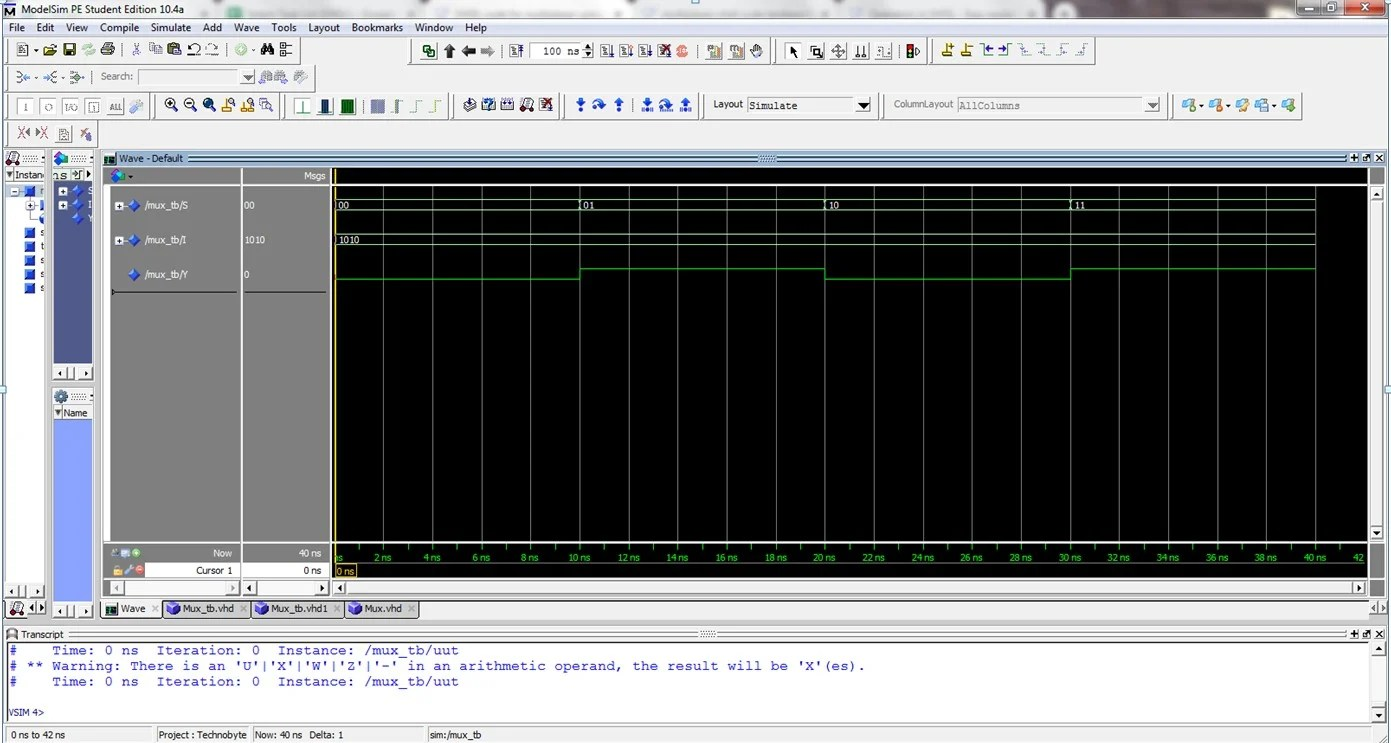View Course Path

# VHDL code for multiplexer using behavioral method – full code and explanation

In this post, we will take a look at implementing the VHDL code for a multiplexer using the behavioral architecture method. Any digital circuit’s truth table gives an idea about its behavior. First, we will take a look at the truth table of the 4×1 multiplexer and then the syntax. We will also write a testbench to verify our code. Then we will generate the RTL schematic and the simulation waveforms. For the full code, scroll down.

Contents

## Explanation of the VHDL code for multiplexer using behavioral method. How does the code work?

A multiplexer is a data selector. It has multiple inputs, out of which it selects one and connects it to the output. This selection is made based on the values of the select inputs.

In this program, we will write the VHDL code for a 4:1 Mux. A 4:1 mux will have two select inputs. Since we are using behavioral architecture, it is necessary to understand and implement the logic circuit’s truth table.

### Truth table of a 4:1 Mux

 I0 I1 I2 I3 S0 S1 Y I0 x x x 0 0 I0 x I1 x x 0 1 I1 x x I2 x 1 0 I2 x x x I3 1 1 I3

The I/O ports of the multiplexer will be vector entities as we are going to code in the truth table. Let’s name our entity as `MUX_SOURCE` and write the syntax for the entity-architecture pair. Note the two `begin` statements with the `process` statement in between as is customary with behavioral architecture in VHDL.

```entity MUX_SOURCE is
Port ( S : in  STD_LOGIC_VECTOR (1 downto 0);
I : in  STD_LOGIC_VECTOR (3 downto 0);
Y : out STD_LOGIC);
end MUX_SOURCE;

architecture Behavioral of MUX_SOURCE is

begin

process (S,I)

begin
```

We will code the behavior of the circuit using the if-elsif statements that are available to us in the behavioral architecture. We have seen on multiple occasions in this VHDL course that the benefit of using the if-elsif statements versus the if-else statements is that you have to use only one closing statement for the entire command. So you can encode multiple `if` statements and have to remember to use only one `end if` statement at the end of the VHDL program. The syntax for coding in the truth table is shown below:

```if (S <= "00") then
Y <= I(0);
elsif (S <= "01") then
Y <= I(1);
elsif (S <= "10") then
Y <= I(2);
else
Y <= I(3);
```

## Full VHDL code for multiplexer using behavioral method

```library IEEE;
use IEEE.STD_LOGIC_1164.ALL;
use IEEE.STD_LOGIC_ARITH.ALL;
use IEEE.STD_LOGIC_UNSIGNED.ALL;

entity MUX_SOURCE is
Port ( S : in  STD_LOGIC_VECTOR (1 downto 0);
I : in  STD_LOGIC_VECTOR (3 downto 0);
Y : out STD_LOGIC);

end MUX_SOURCE;

architecture Behavioral of MUX_SOURCE is

begin

process (S,I)

begin

if (S <= "00") then
Y <= I(0);
elsif (S <= "01") then
Y <= I(1);
elsif (S <= "10") then
Y <= I(2);
else
Y <= I(3);

end if;
end process;
end Behavioral;

```

## Testbench for 4×1 Mux using VHDL

We will be using a testbench with a process statement. You can check out the different types of testbenches in VHDL here.

```library ieee;
use ieee.std_logic_1164.all;

entity mux_tb is
end entity;

architecture tb of mux_tb is
component MUX_SOURCE is
Port ( S : in STD_LOGIC_VECTOR (1 downto 0);
I : in STD_LOGIC_VECTOR (3 downto 0);
Y : out STD_LOGIC);
end component;

signal S : STD_LOGIC_VECTOR(1 downto 0);
signal I : STD_LOGIC_VECTOR(3 downto 0);
signal Y : STD_LOGIC;

begin
uut : MUX_SOURCE port map(
S => S,
I => I,
Y => Y);

stim : process
begin

I(0) <= '0';
I(1) <= '1';
I(2) <= '0';
I(3) <= '1';

S <= "00";wait for 10 ns;
S <= "01";wait for 10 ns;
S <= "10";wait for 10 ns;
S <= "11";wait for 10 ns;
wait;
end process;
end tb;```

## RTL schematic## Simulation waveform for 4×1 MuxWere you able to encode your multiplexer? Let us know what you did differently by commenting below. Also, would you like us to include the VHDL code for an 8:1 mux?

Edit: Post updated with the testbench, RTL Schematic, and Simulation Waveform by Deepak Joshi.

## 2 thoughts on “VHDL code for multiplexer using behavioral method – full code and explanation”

1.Subham Ghosh says:

can you also show the VHDL test bench code

1.Umair Hussaini says:

Alright! We’ll update this post with the testbench and will mail you an update when we do!

This site uses Akismet to reduce spam. Learn how your comment data is processed.